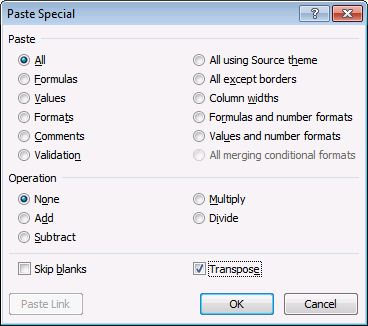Author: Oscar Cronquist Article last updated on February 10, 2023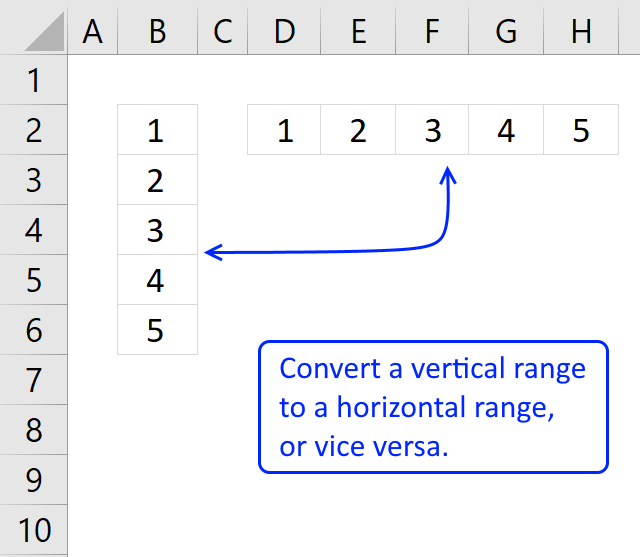The TRANSPOSE function allows you to convert a vertical range to a horizontal range, or vice versa.

A vertical range is a range with values in one column, a horizontal range has values in one row.

You can also transpose a range with more than one column and one row.

Array formula in cell D2:H2:

=TRANSPOSE(B2:B6)

Range B2:B6 has 1 column and 5 rows and it is a vertical range. Use the TRANSPOSE function to convert it to a horizontal range. Shown in the example above.

TRANSPOSE(array)

### Arguments

 array The values you want to transpose.

=TRANSPOSE(B2:B6) is entered in cell range D2:H2, as an array formula.

## 1. How to enter an array formula1. Select cell range D2:H2.
2. Press with left mouse button on in the formula bar.
3. Type: =TRANSPOSE(B2:B6) in the formula bar.
4. Press and hold CTRL + SHIFT simultaneously.
5. Press Enter once.
6. Release CTRL + SHIFT.

If you did this right the formula now begins and ends with a curly bracket. Like this: {=TRANSPOSE(B2:B6)} Don't enter these characters yourself.

### 1.1 Why do I have to enter the TRANSPOSE function as an array formula?

It returns more than one value, you need to enter it as an array formula if you want to see all values on the worksheet.

### 1.2 Excel 365 users can enter the TRANSPOSE function as a regular formula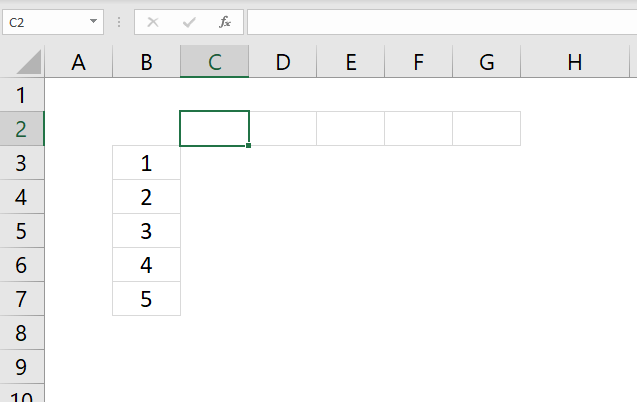Excel 365 users can now enter dynamic array formulas as regular formulas. Dynamic array formulas expand automatically, this is called spilling.

## 2. What happens if you transpose a range with the same number of columns and rows?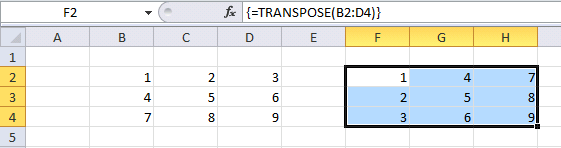The picture shows what happens if you transpose a cell range with the same number of rows and columns, in this case, a cell range with 3 columns and 3 rows.

The range size doesn't change but the numbers in the range change positions. The values in the first column (B2:B4) is converted into the first row (F2:H2), the same with the second column (C2:C4) into the second row (F3:H3), and so on.

## 3. How to converting a vertical cell range to constants

This animated picture shows how to convert the values in a cell range to constants.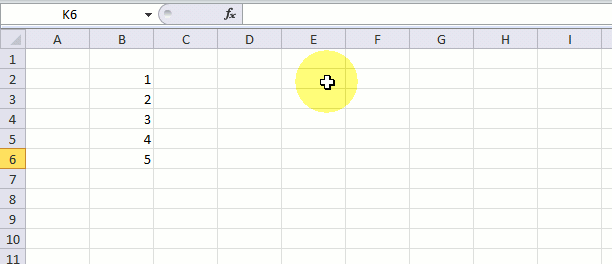What the animated picture doesn't show you is that I am pressing F9 after selecting the cell range B2:B6. These are all the steps:

1. Select cell D2.
2. Type =
3. Select cell range B2:B6.
4. Press F9.

The formula bar returns {1;2;3;4;5}. The curly brackets tell you that it is an array. The constants in the array are separated by semicolons. The semicolon tells you that the next value is in the row below. In the example above all values are separated vertically, this is a one-dimensional array.

## 4. Transpose a vertical array with constants

What happens if we transpose this array? {1;2;3;4;5}  Select a cell and type in formula bar: =TRANSPOSE({1;2;3;4;5}) Press F9.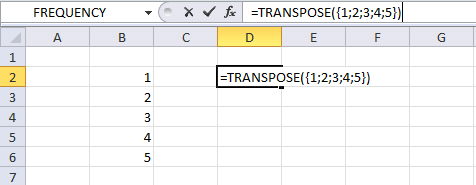The formula bar shows {1,2,3,4,5}. The comma tells you that the values in the array are separated horizontally.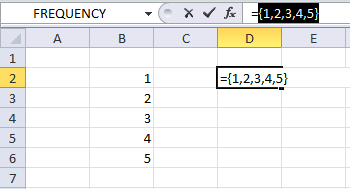You can verify this by selecting D2:H2, type =TRANSPOSE({1;2;3;4;5}) in the formula bar and enter it as an array formula.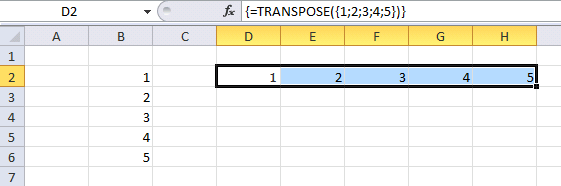In other words, the colon tells you that the next value in the array is in the next column. The semicolon tells you that the next value is in the row below.

## 5. Two-dimensional arrays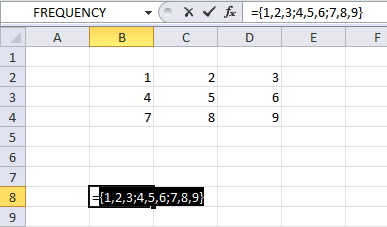Look at this array {1,2,3;4,5,6;7,8,9}. You can now distinguish that this array has 3 columns and 3 rows by looking at the commas and semicolons. The array has values in more than one column and one row. This is a two-dimensional array.

I made this array {1,2,3;4,5,6;7,8,9} from cell range B2:D4, it has 3 columns and 3 rows.